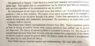# On soundness and completeness of ZFC set theory

schniefen
Homework Statement: See attached image.
Homework Equations: ZFC set theory.

Consider the text in the attached image. What is meant with "We require of an axiom system that it be possible to decide whether or not any given formula is an axiom."? Is consistency synonymous with soundness? Is there an axiom system that can be completely and consistently axiomatised, or is this what Gödel's incompleteness theorem states is not possible?

#### Attachments

•IMG_3137.jpg
101.7 KB · Views: 202

Gold Member
I think the statement is you want to decided whether a wff is a theorem, not an axiom. The axioms are chosen. Godel's incompleteness says an axiom system of certain "richness" or complexity that includes number theory or Peano arithmetic cannot prove its own consistency, i.e., you must step outside of it to prove its consistency. Soundness in deductive systems is the same as valid: an argument is valid if when the premises are true, the conclusion cannot be false. Consistency means you cannot derive a statement and its negation within the system. It can be explicit as in having an axiom and its negation in the axiom system or not so explicit in that you may derive a wff and its negation from within the system. Maybe @SSequence can give a more detailed answer?

Last edited:
•sysprog
schniefen
Is it due to Gödel’s theorem that set theory can’t be completely and consistently axiomatised? What would this mean in more simple language? And what is the difference between soundness and consistency in this case? (If the definition of soundness is that one can’t prove anything that’s false, consistency seems like a synonymous notion.)

Gold Member
Edit:Many use it interchangeably. A collection of statements is consistent if they can all be simultaneously true and for a formal system, if no contradiction can be deduced from the axioms. Truth here will require an interpretation /semantics. Re Godel, the theorem just says that systems that can model number theory cannot prove their own consistency. Beyond certain complexity, axiom systems cannot prove their own consistency. So set theory is not part of the scope of the theorem. Ultimately every theory is axiomatizable in that you can make each formula into an axiom ( assuming consistency).

Last edited:
SSequence
Maybe @SSequence can give a more detailed answer?
@stevendaryl is more knowledgeable on these matters (than me). But he doesn't seem to have logged-in for while.

•WWGD
Gold Member
What is meant with "We require of an axiom system that it be possible to decide whether or not any given formula is an axiom."?
I think the relevant portion of the text comes immediately after that quote:
"Under that assumption, set theory cannot be completely and consistently axiomatized."

The reason is because of the axiom schema of induction. This is an axiom schema, rather than an axiom, so there are in reality an infinite number of axioms of set theory. I'd never thought about it exactly this way, but I suppose this means that no formal procedure can exist to decide whether any particular formula is an axiom (falling out from the axiom schema of induction).

Is consistency synonymous with soundness?
They're certainly very closely related, but I would probably say no, because soundness explicitly deals with the link between semantic and syntactic entailment. In simple terms, a logical system is sound iff for any theorem derivable from the set of axioms, that theorem is valid in all interpretations of the system.

On the other hand, consistency merely means that one cannot derive both ##p## and ##\neg p##. This can be viewed either syntactically or semantically, but there is no implication of a link between the two.

It's a subtle difference at best, and in practice, I'm not sure it matters. I suppose there may be some pathological situation where a system is unsound (can prove a falsehood) but not inconsistent (cannot prove ##p \wedge \neg p## in particular). But I'm not even sure that's possible.

Is there an axiom system that can be completely and consistently axiomatised
Propositional and predicate logic are both consistent and complete. Here's a reddit thread I found that mentions a number of other systems:

AplanisTophet
To give a more direct answer to your question, axioms are presumed true. If you can then prove things (theorems) as true given your axioms, the distinction between axioms and theorems when it comes to their truth values is trivial. All theorems could be made axioms if they are provable from your original (smaller) set of axioms and the models that adhere to the axioms / theorems are the same (I believe... I'm an amateur generally).